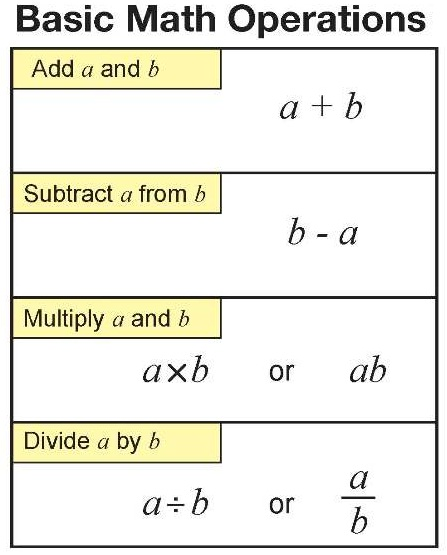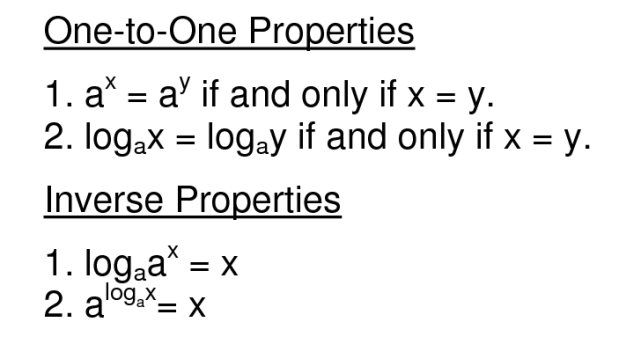# Basic mathematical operations

This section deals with the basic mathematical operations and their application, as well as some operations that are derived from the basics, but are invaluable as mathematical tools or as the foundation for understanding more complex mathematics.

## Types of basic mathematical operations

There are multiple views to which mathematical operations can be considered “basic”. The general consensus is that the basic mathematical operations are: addition, subtraction, multiplication and division. Counting can also be considered as a member of that group, but more often it is thought of as a form of addition because it comes down to adding one to the previous value until you have counted every object you intended to count.There are also more complex operations that can be thought of as fundamental in a way. They are operations with percentages, square roots, exponentiation and logarithmic functions. Percentages are a way of expressing a number as a fraction of 100. It is extremely useful to know your way around them since they are a piece of mathematics we encounter every single day. Square roots are used everywhere from simple geometry to more advanced mathematics, as well as statistics. Exponentiation, as a form of repeated multiplication, is also present in all fields of science and life – whether it is economics, biology, chemistry, physics or something completely different. Logarithmic functions are also considered to be one of the basic mathematical operations since they are a kind of a reverse operation of exponentiation. They also have a large range of applications in different contexts and surroundings.Also, in this section we will go through the order of operations, which is one of the fundamental rules in mathematics that helps maintain consistency in writing and solving mathematical expressions.

Of course, you can also expect a large number of worksheets to accompany each and every lesson in order to help your learning process and to make basic mathematical operations easier to understand.